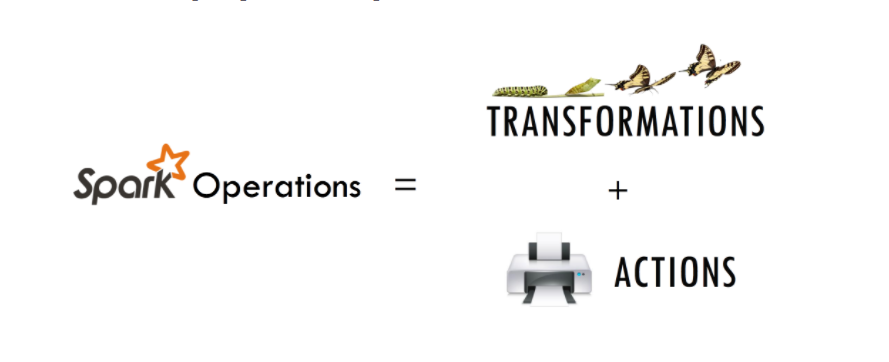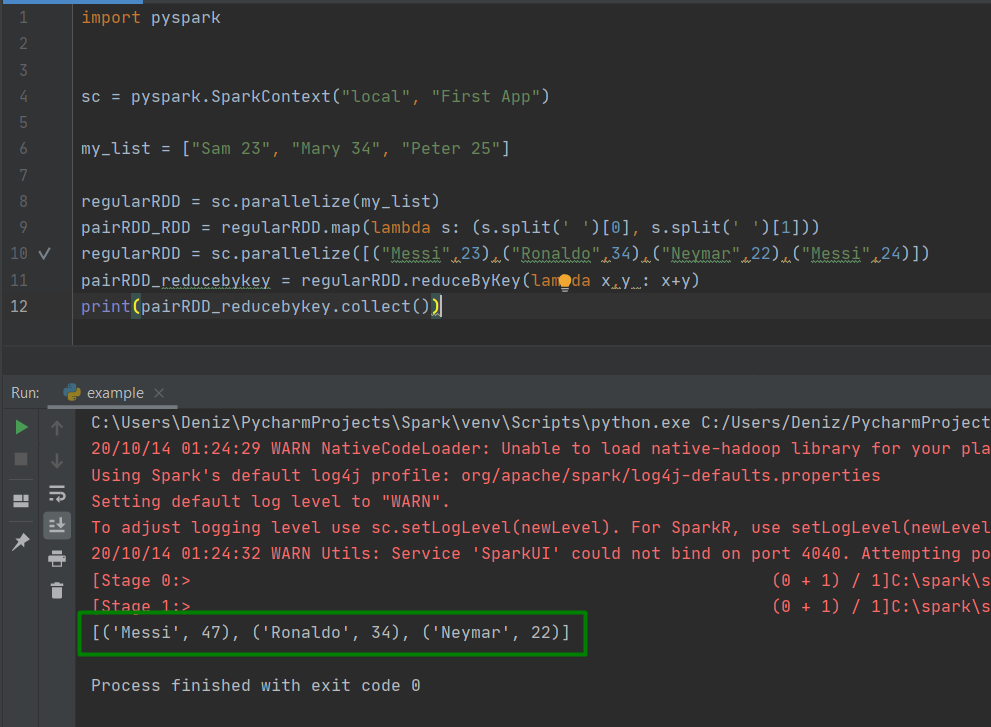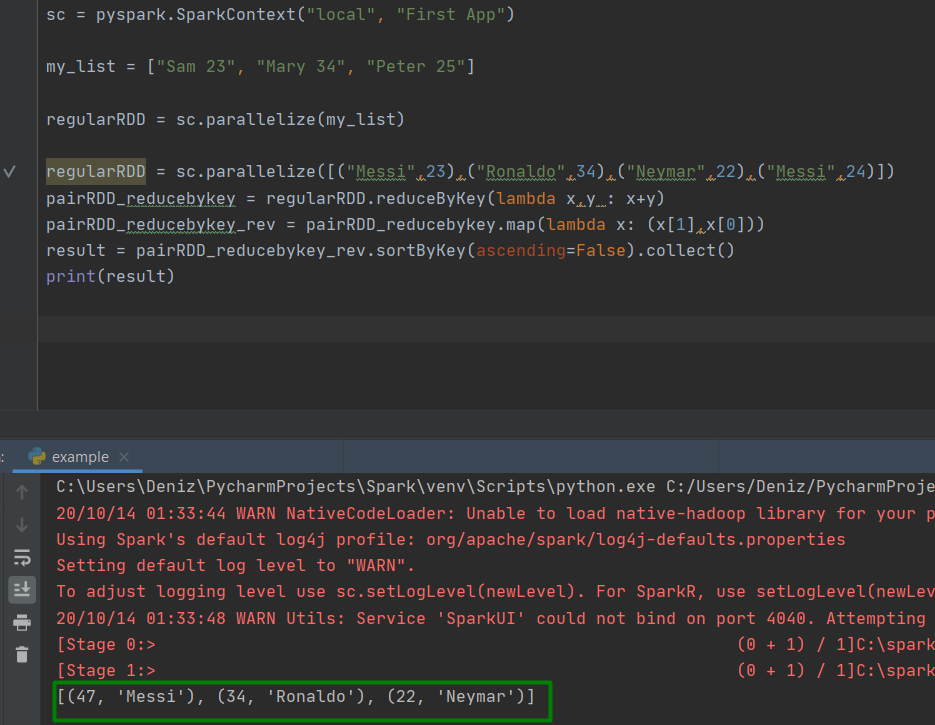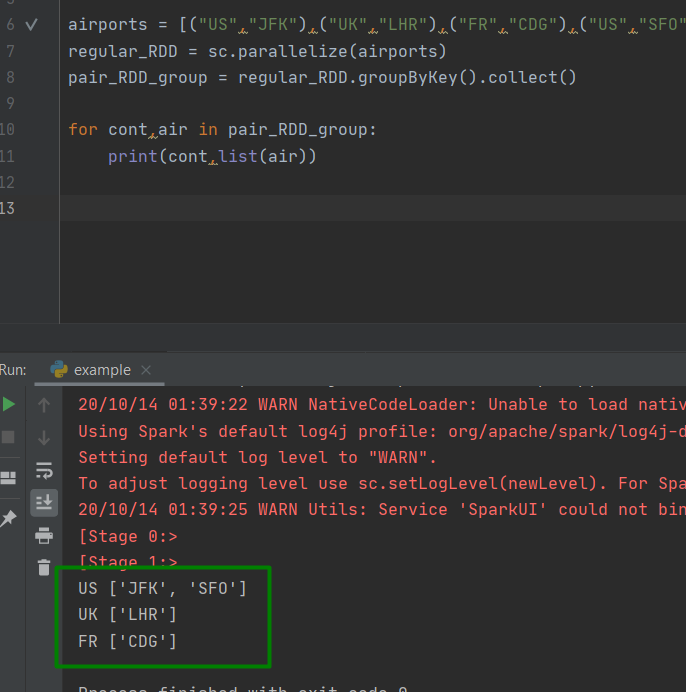# Introduction to PySpark RDD

In this chapter, we will start with RDDs which are Spark’s core abstraction for working with data.

### What is RDD

• RDD = Resilient Distributed Datasets

it’s simply collection of data distributed across the cluster, RDD is the fundamental and backbone data type in PySpark.

#### Decomposing RDDs

The name RDD captures 3 important properties.

• Resilient Distributed Datasets
• Resilent: Ability to withstand failures
• Distributed: Spanning across multiple machines
• Datasets : Collection of partitioned data e.g, Arrays, Tables, Tuples, etc.

#### Creating RDDs

• Parallelizing an existing collection of objects
• External datasets:
• Files in HDF
• Objects in Amazon S3 bucket
• lines in a text file
• From existing RDDs

## RDD Operations in PySpark

in PySpark supports two different types of operations Transformations and Actions.  Transformations are operations on RDDs that return a new RDD and Actions are operations that perform some computation on the RDD.• Basic RDD Transformations
• map() , filter() flatMap() , and union()### Example

```# Create map() transformation to cube numbers
numbRDD = sc.parallelize(range(1,10))
cubedRDD = numbRDD.map(lambda x: x ** 3)

# Collect the results
numbers_all = cubedRDD.collect()

# Print the numbers from numbers_all
for numb in numbers_all:
print(numb)```Pair RDD in PySpark

• Real life datasets are usually key/value pairs
• Each row is a key and maps to one or more values
• Pair RDD is a special data structure to work with this kind of dataset
• Pair RDD: key is the identifier and value is data

Let’s give an example

### reducebykey()

```import pyspark

sc = pyspark.SparkContext("local", "First App")

my_list = ["Sam 23", "Mary 34", "Peter 25"]

regularRDD = sc.parallelize(my_list)
pairRDD_RDD = regularRDD.map(lambda s: (s.split(' '), s.split(' ')))
regularRDD = sc.parallelize([("Messi",23),("Ronaldo",34),("Neymar",22),("Messi",24)])
pairRDD_reducebykey = regularRDD.reduceByKey(lambda x,y : x+y)
print(pairRDD_reducebykey.collect())```### sortByKey()

```pairRDD_reducebykey_rev = pairRDD_reducebykey.map(lambda x: (x,x))
result = pairRDD_reducebykey_rev.sortByKey(ascending=False).collect()
print(result)
```### groupByKey()

```airports = [("US","JFK"),("UK","LHR"),("FR","CDG"),("US","SFO")]
regular_RDD = sc.parallelize(airports)
pair_RDD_group = regular_RDD.groupByKey().collect()

for cont,air in pair_RDD_group:
print(cont,list(air))
```See you in the next article.

6,983 views last month,  1 views today